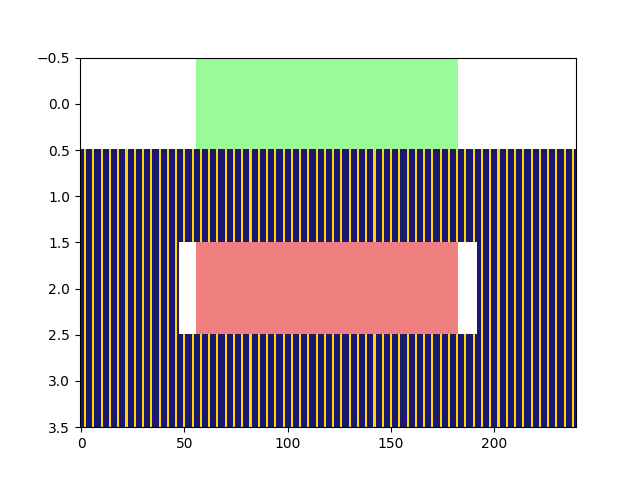# Synchronization Signal Block (SSB) Grid Generation

THe modules maps the primary synchronization signal (PSS), secondary synchronization signal (SSS), demodulation reference signal (DMRS) for PBCH and PBCH payload to SSB grid. The SSB grid in 5G spans 4 OFDM symbols and 240 subcarriers (12 RBs). This modules can return multiple SSBs carrying same PSS, SSS but DMRS and different PBCH information which is typically the case when transmitting multiple SSBs in a half frame. In such cases, only the SSB index information changes across SSBs. The shape of the variable is shown in table-1.

Important

The cell-ID ($$\text{N}_{\text{ID}}$$), half frame index ($$\text{n}_{\text{HF}}$$) and maximum number of SSBs in a half frame ($$\text{L}_{\text{max}}$$) should be same for all the components of SSB.

Table 6 Table-1: Sizes of the components of SSBs

Dimension

Primary synchronization signal (PSS)

$$1 \times 127$$

Secondary synchronization signal (PSS)

$$1 \times 127$$

Demodulation reference signal (DMRS)

$$\text{N}_{\text{SSBs}} \times 144$$

$$\text{N}_{\text{SSBs}} \times 432$$

When $$\text{N}_{\text{SSBs}} = 1$$:

numSSBs      = 1
N_ID1        = np.random.randint(336)
N_ID2        = np.random.randint(3)
N_ID         = 3*N_ID1 + N_ID2

nssbCandidatesInHrf = int(np.random.choice([4,8,64]))
ssbIndex            = np.random.choice(nssbCandidatesInHrf, size = numSSBs)
hrfBit              = 0

# Generate PSS sequence
pssObject    = PSS(N_ID2);
pssSequence  = pssObject()

# Generate SSS sequence
sssObject    = SSS(N_ID1, N_ID2);
sssSequence  = sssObject()

# Generate DMRS sequence
dmrsLen      = 144;
dmrsObject   = DMRS("PBCH", N_ID, ssbIndex, nssbCandidatesInHrf, hrfBit)

# Symbol mapping object
mapper       = Mapper("qam", 2)
pbchSymbols  = mapper(np.random.randint(2, size = (numSSBs, 864))) # Generate symbols from the bits.

# Generate SSB Grid
ssbObject    = SSB_Grid(N_ID)
ssb          = ssbObject(pssSequence, sssSequence, dmrsSequence, pbchSymbols) # Generate SSB Grid of shape (1, 4, 240)

# Display the location of PSS, SSS, DMRS and PBCH in SSB
ssbObject.displayGrid(option=1)When $$\text{N}_{\text{SSBs}} > 1$$ :

numSSBs      = 10
N_ID1        = np.random.randint(336)
N_ID2        = np.random.randint(3)
N_ID         = 3*N_ID1 + N_ID2

nssbCandidatesInHrf = int(np.random.choice([4,8,64]))
ssbIndex            = np.random.choice(nssbCandidatesInHrf, size = numSSBs)
hrfBit              = 0

# Generate PSS sequence
pssObject    = PSS(N_ID2);
pssSequence  = pssObject()

# Generate SSS sequence
sssObject    = SSS(N_ID1, N_ID2);
sssSequence  = sssObject()

# Generate DMRS sequence
dmrsLen      = 144;
dmrsObject   = DMRS("PBCH", N_ID, ssbIndex, nssbCandidatesInHrf, hrfBit)

# Symbol mapping object
mapper       = Mapper("qam", 2)
pbchSymbols  = mapper(np.random.randint(2, size = (numSSBs, 864))) # Generate symbols from the bits.

# Generate SSB Grid
ssbObject    = SSB_Grid(N_ID)
ssb          = ssbObject(pssSequence, sssSequence, dmrsSequence, pbchSymbols) # Generate SSB Grid of shape (10, 4, 240)
# Display the location of PSS, SSS, DMRS and PBCH in SSB
ssbObject.displayGrid(option=0)More example on SSB Resource mapping can be found in Tutorials. The details about the input-output interface of the SSB_Grid implementation is provided below.

class toolkit5G.ResourceMapping.SSB_Grid(N_ID, isNormalized=False)[source]

Generates the Synchronization signal block grid as per Section 7.4.3 [3GPPTS38211_SSBGrid].

Parameters:
• N_ID (int) – Defines the cell-ID $$\in \{0,1,...,1007\}$$.

• isNormalized (bool) – Defines the normalization flag. This flag is used to normalize the power of each symbol in SSB to one.

Important

If SSB is un-normalized, the total power is different across symbols.

Input:
• pssSeq ([127,], np.complex64) – Defines PSS Sequence of length 127 to be loaded in the SSB.

• sssSeq ([127,], np.complex64) – Defines SSS Sequence of length 127 to be loaded in the SSB.

• dmrsSeq ([:, 144], np.complex64) – Defines DMRS Sequence of length 144 to be loaded in the SSB.

• pbchSymb ([:, 432], np.complex64) – Defines PBCH Payload of length 432 to be loaded in the SSB. It shouldn’t have maore than 2 dimensions.

Warning

pbchSymb and dmrsSeq shouldn’t have more than 2 dimensions. Moreover, both these variables should have same number of SSBs (pbchSymb.shape should equal dmrsSeq.shape).

Important

Cell-ID should be same for all the pbchSymb and dmrsSeq .

Output:

[…, 4,240], np.complex64 – Synchronization signal block grid.

Raises:
• Exception – [Error-SSB_Grid]: Cell-ID can not be larger than 1007!

• Exception – [Error-SSB_Grid]: Cell-ID can not take a negative value!

• Exception – [Error-SSB_Grid]: length of PSS-indices not equal to 127!

• Exception – [Error-SSB_Grid]: length of SSS-indices not equal to 127!

• Exception – [Error-SSB_Grid]: Indices must be a 2D tuple with length equal to 432!

• Exception – [Error-SSB_Grid]: length of DMRS-indices not equal to 144!

• ValueError – [Error-SSB_Grid]: Index exceeded total number of SSBs= numSSBs!

property dmrsIndices

Defines the 2D Indices (2,432) where DMRS sequences are loaded in each SSB.

property pbchIndices

Defines the 2D Indices (2,432) where PBCH Payloads are loaded in each SSB.

property pssIndices

Defines the 2D Indices (2,127) where PSS are loaded in each SSB.

property sssIndices

Defines the 2D Indices (2,127) where SSS are loaded in each SSB.

SSB_Grid.displayGrid(index=0, option=1, displayGrid=True)[source]

Provides the method to display SSB grid.

Input:
• index (int) – Define the Index of SSB whose grid has to be displayed.

• option (int $$\in \{0,1\}$$.) –

• If option=0 then heat map of the SSB is displayed.

• If option=1 then displays the grid locations (relative t-f locations) where PSS, SSS, DMRS and PBCH payload are loaded in the SSB grid.

References:

(3GPP TS 38.211 version 17.1.0 Release 17) 5G;NR;Physical channels and modulation ‘5.2.2 Low-PAPR sequence generation type 1’